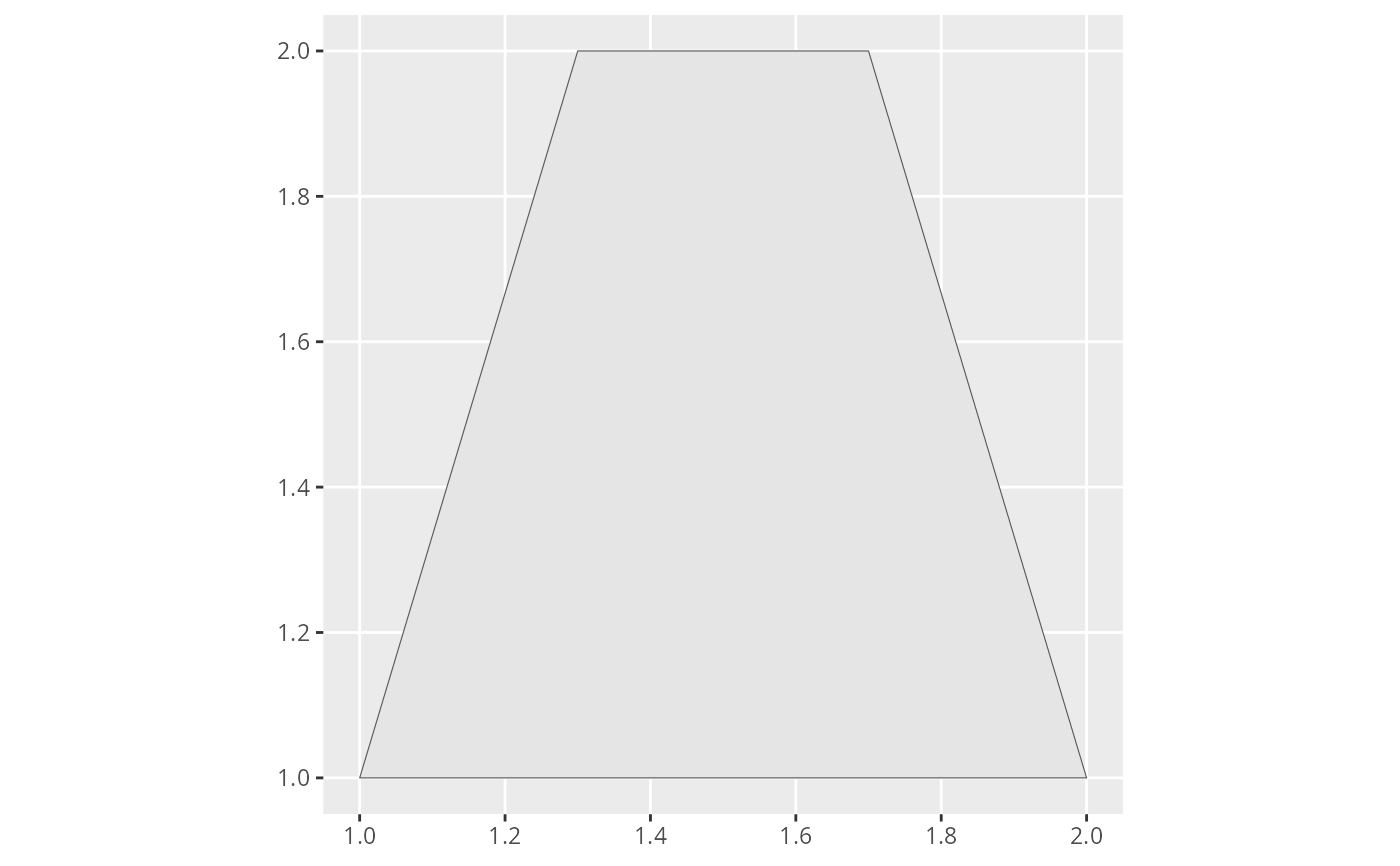A polygon can be described as a sequence of points defining the polygon's boundary. When given such a sequence (anti clockwise!) this function creates a SpatialPolygonsDataFrame holding the polygon decribed. By default, the first two columns of data are assumed to define the x and y coordinates of the points. This behavior can ba changed using the cols parameter, which points out the names of the columns holding the coordinates. The coordinate reference system of the resulting spatial polygon can be set via the crs paraemter. Posterior conversion to a different CRS is supported using the to.crs parameter.

## Usage

spoly(data, cols = colnames(data)[1:2], crs = fm_CRS(), to.crs = NULL)

## Arguments

data

A data.frame of points describing the boundary of the polygon

cols

Column names of the x and y coordinates within the data

crs

Coordinate reference system of the points

to.crs

Coordinate reference system for the SpatialLines ouput.

## Value

SpatialPolygonsDataFrame

## Examples

# \donttest{
# Create data frame of boundary points (anti clockwise!)
pts <- data.frame(
x = c(1, 2, 1.7, 1.3),
y = c(1, 1, 2, 2)
)

# Convert to SpatialPolygonsDataFrame
pol <- spoly(pts)

if (require(ggplot2, quietly = TRUE) &&
require(ggpolypath, quietly = TRUE)) {
# Plot it!
ggplot() +
gg(pol)
}
#> Regions defined for each Polygons# }